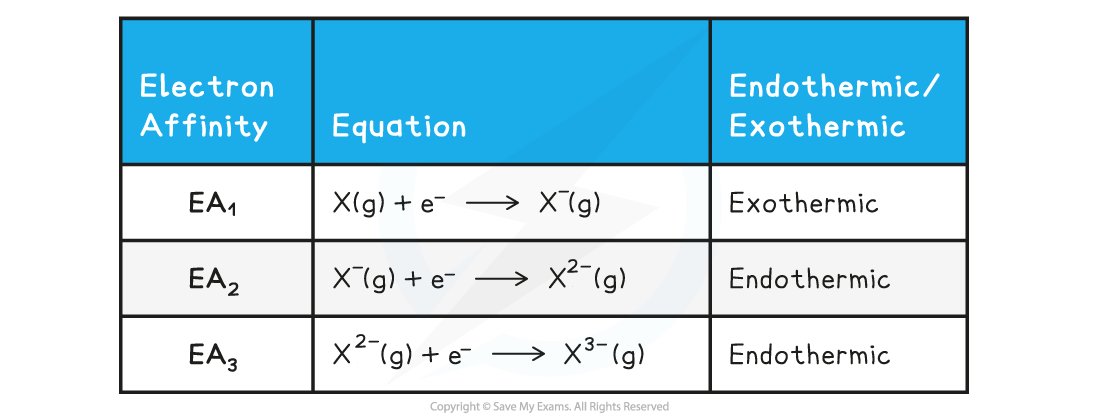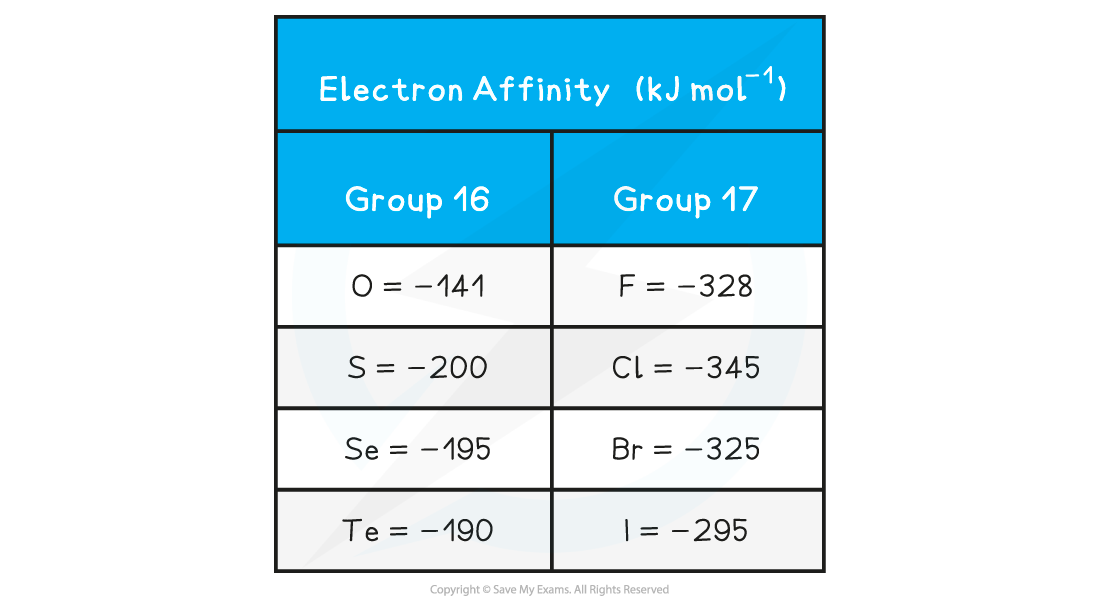# CIE A Level Chemistry复习笔记5.1.2 Electron Affinity & Trends of Group 16 & 17 Elements

### Electron Affinity

#### Electron affinity

• The first electron affinity (EA1) is the enthalpy change when 1 mole of electrons is added to 1 mole of gaseous atoms, to form 1 mole of gaseous ions each with a single negative charge under standard conditions

X(g) + e- → X-(g)

• EA1 is usually exothermic, as energy is released
• Since this is generally an exothermic process, then the value for EAwill usually be a negative number
• An element can also accept more than one electron, in which case successive electron affinities are used
• For example, the second electron affinity (EA2) and third electron affinity (EA3) of an element represent the formation of 1 mole of gaseous ions with 2- and 3- charges respectively
• The second and third electron affinities are endothermic, as energy is absorbed
• This is because the incoming electron is added to an already negative ion
• Energy is required to overcome the repulsive forces between the incoming electron and negative ion
• Since these are endothermic processes, the values will be positive

Second & third electron affinity table#### Factors affecting electron affinity

• The value of the electron affinity depends on how strongly the incoming electron is attracted to the nucleus
• The greater the attractive forces between the electron and nucleus, the more energy is released and therefore the more exothermic (more negative) the EA1 value will be
• The factors affecting the electron affinity of an element are the same as those that affect the ionisation energy (the formation of positive ions via the loss of electrons)
• These are:
• Nuclear charge: the greater the nuclear charge, the stronger the attractive forces between an incoming electron and the nucleus
• Distance: the greater the distance between the nucleus and the outermost shell/orbital where the electron is added, the weaker the force of attraction
• Shielding: the greater the number of shells, the greater the shielding effect and the weaker the force of attraction

#### Trends in electron affinity of Group 16 & Group 17 elements

• Electron affinities of non-metals become more exothermic across a period, with a maximum at Group 17
• There is generally a downwards trend in the size of the electron affinities of the elements in Group 16 and 17
• The electron affinities generally become less exothermic for each successive element going down both Groups, apart from the first member of each Group (oxygen and fluorine respectively)

Electron affinity table• An atom of chlorine has a greater nuclear charge than an atom of sulfur
• Chlorine will therefore have a greater attractive force between its nucleus and its outer electrons
• More energy is released upon adding an electron to chlorine, so the EA1of Cl is more exothermic than for S
• Going down Group 16 and 17:
• The outermost electrons are held less tightly to the nucleus as they are further away
• The number of electron shells increases causing an increased shielding of the outermost electrons
• It gets more difficult to add an electron to the outer shell
• Less energy is released upon adding an electron to the outer shell
• So generally, the EA1becomes less exothermic
• Fluorine is an exception and has a lower EA1 than chlorine
• Fluorine has a very small atomic radius
• This means that the electron density of fluorine is high
• There is more repulsion between the incoming electron and the electrons that are already present in fluorine
• These repulsive forces reduce the attractive forces between the incoming electron and nucleus
• As a result, the EA1 of fluorine is less exothermic than expected